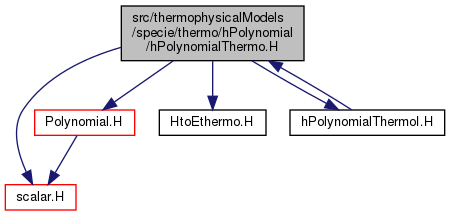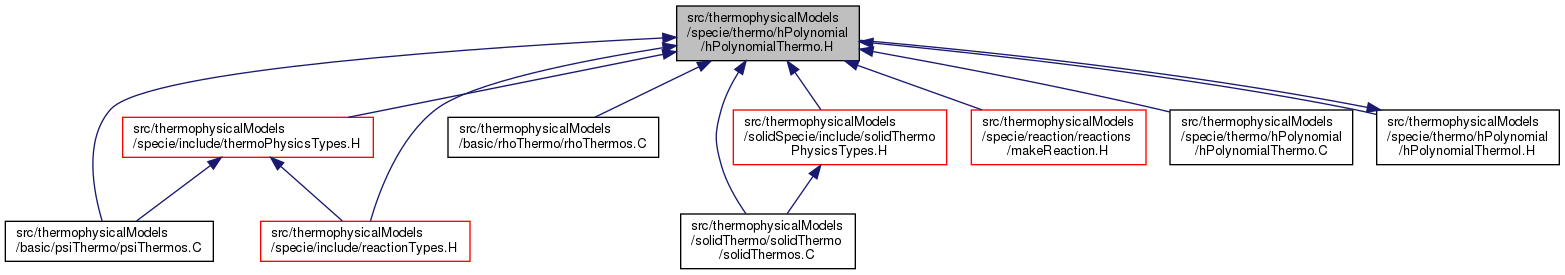The OpenFOAM FoundationhPolynomialThermo.H File Reference
Include dependency graph for hPolynomialThermo.H:This graph shows which files directly or indirectly include this file:Go to the source code of this file.

## Classes

class  hPolynomialThermo< EquationOfState, PolySize >
Thermodynamics package templated on the equation of state, using polynomial functions for `cp`, `h` and `s`. More...

class  hPolynomialThermo< EquationOfState, PolySize >
Thermodynamics package templated on the equation of state, using polynomial functions for `cp`, `h` and `s`. More...

## Namespaces

Foam
Namespace for OpenFOAM.

## Functions

template<class EquationOfState , int PolySize>
hPolynomialThermo< EquationOfState, PolySize > operator+ (const hPolynomialThermo< EquationOfState, PolySize > &, const hPolynomialThermo< EquationOfState, PolySize > &)

template<class EquationOfState , int PolySize>
hPolynomialThermo< EquationOfState, PolySize > operator* (const scalar, const hPolynomialThermo< EquationOfState, PolySize > &)

template<class EquationOfState , int PolySize>
hPolynomialThermo< EquationOfState, PolySize > operator== (const hPolynomialThermo< EquationOfState, PolySize > &, const hPolynomialThermo< EquationOfState, PolySize > &)

template<class EquationOfState , int PolySize>
Ostream & operator<< (Ostream &, const hPolynomialThermo< EquationOfState, PolySize > &)

## Detailed Description

Original source file hPolynomialThermo.H

Definition in file hPolynomialThermo.H.